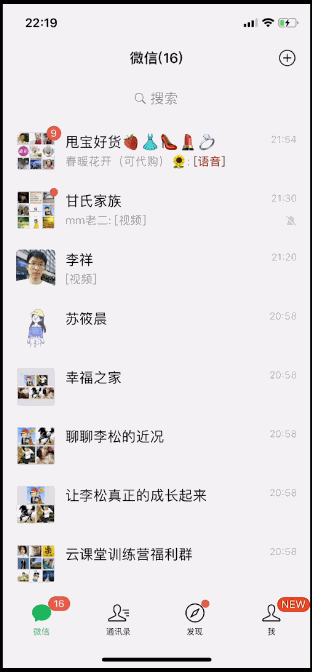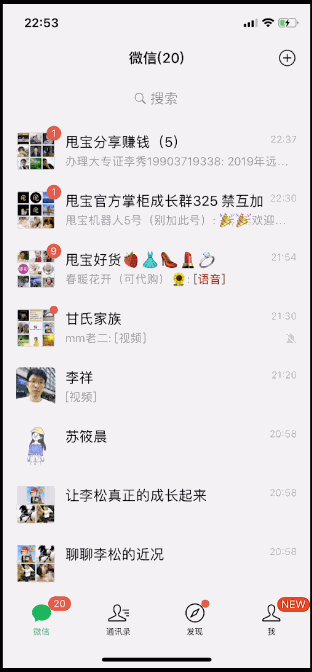# 置顶已上线小程序，在IOS端无法调起wx.scanCode，本地IOS、安卓均正常！精选热门

- 当前 Bug 的表现（IOS端，进入线上版本的小程序△线上版本演示

- 预期表现（IOS端，进行本地调试小程序△本地调试演示

 `onLoad: ``function` `() {``  ``var` `that = ``this``;``  ``// 真实数据————开始``  ``setTimeout (``function` `() {``    ``wx.scanCode({``      ``success(res) {``        ``//扫书成功 ISBN``        ``console.log(res);`` ` `        ``wx.cloud.callFunction({``          ``// 云函数名称``          ``name: ``'getBook'``,``          ``// 传给云函数的参数``          ``data: {``            ``ISNB: res.result``          ``},``          ``success: ``function` `(res) {``            ``console.log(``'获取云端书籍信息成功'``, res);``            ``try` `{``              ``//将JSON字符串，转化为对象。``              ``res.result = JSON.parse(res.result);``            ``} ``catch` `(ex) {``              ``res.result = {};``            ``}``            ``that.setData({``              ``showPage: ``true``,``              ``info: res.result,``              ``// info: that.dealData(res.result),              ``            ``})``          ``},``          ``fail: ``function` `() {``            ``console.log(``"fail"``)``          ``}``        ``})``      ``},``      ``fail: ``function` `() {``        ``// 如果扫书失败``        ``wx.navigateTo({``          ``url: ``'/pages/list/list'``,``        ``});``      ``}``    ``});``  ``}, 500)``  ``// 真实数据————结束``},`

1 个回答